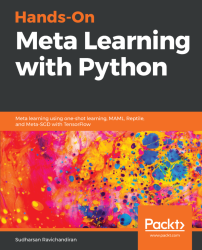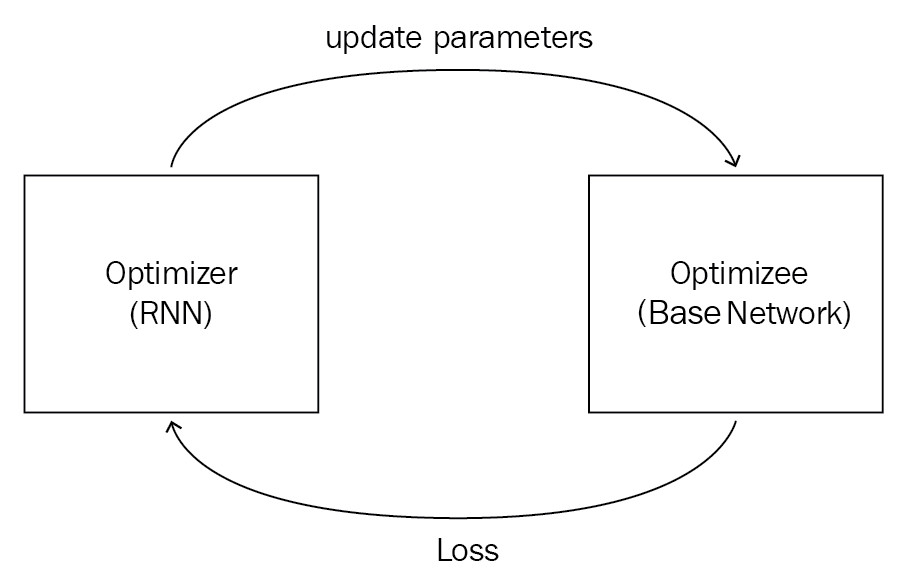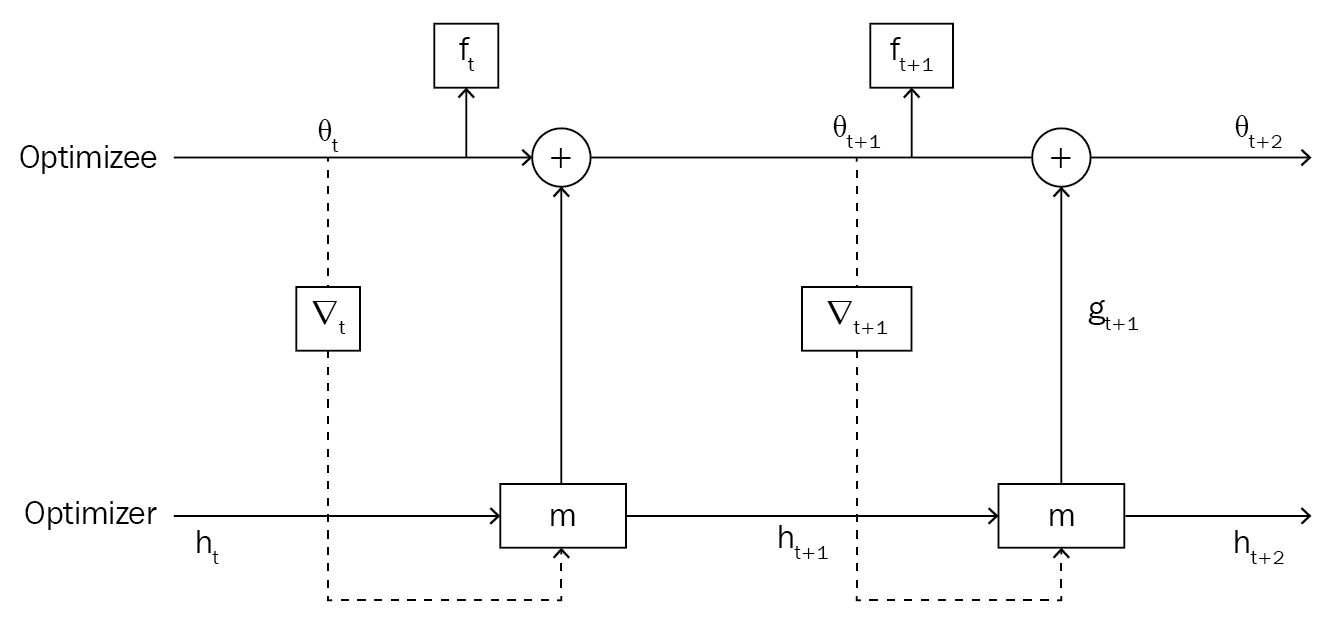•#### Hands-On Meta Learning with Python#### Overview of this book

Meta learning is an exciting research trend in machine learning, which enables a model to understand the learning process. Unlike other ML paradigms, with meta learning you can learn from small datasets faster. Hands-On Meta Learning with Python starts by explaining the fundamentals of meta learning and helps you understand the concept of learning to learn. You will delve into various one-shot learning algorithms, like siamese, prototypical, relation and memory-augmented networks by implementing them in TensorFlow and Keras. As you make your way through the book, you will dive into state-of-the-art meta learning algorithms such as MAML, Reptile, and CAML. You will then explore how to learn quickly with Meta-SGD and discover how you can perform unsupervised learning using meta learning with CACTUs. In the concluding chapters, you will work through recent trends in meta learning such as adversarial meta learning, task agnostic meta learning, and meta imitation learning. By the end of this book, you will be familiar with state-of-the-art meta learning algorithms and able to enable human-like cognition for your machine learning models.
Title PageDedicationContributorsPrefaceFree Chapter
Introduction to Meta LearningFace and Audio Recognition Using Siamese NetworksPrototypical Networks and Their VariantsRelation and Matching Networks Using TensorFlowMemory-Augmented Neural NetworksMAML and Its VariantsMeta-SGD and ReptileGradient Agreement as an Optimization ObjectiveAssessmentsOther Books You May EnjoyIndexNow, we will see one of the interesting meta learning algorithms called learning to learn gradient descent by gradient descent. Isn't the name kind of daunting? Well, in fact, it is one of the simplest meta learning algorithms. We know that, in meta learning, our goal is to learn the learning process. In general, how do we train our neural networks? We train our network by computing loss and minimizing the loss through gradient descent. So, we optimize our model using gradient descent. Instead of using gradient descent can we learn this optimization process automatically?

But how can we learn this? We replace our traditional optimizer (gradient descent) with the Recurrent Neural Network (RNN). But how does this work? How can we replace gradient descent with RNN? If you examine closely, what are we really doing in gradient descent? It is basically a sequence of updates from the output layer to the input layer and we store these updates in a state. So, we can use RNN and store the updates in an RNN cell.

So, the main idea of this algorithm is to replace gradient descent with RNN. But the question is how do RNNs learn? How can we optimize the RNN? For optimizing an RNN, we use gradient descent. So, in a nutshell, we are learning to perform gradient descent through an RNN and that RNN is optimized by gradient descent and that's what is meant by the name learning to learn gradient descent by gradient descent.

We call our RNN, an optimizer and our base network, an optimizee. Let's say we have a modelparameterized by some parameter. We need to find this optimal parameter, so that we can minimize the loss. In general, we find this optimal parameter through gradient descent, but now we use the RNN for finding this optimal parameter. So the RNN (optimizer) finds the optimal parameter and sends it to the optimizee (base network); the optimizee uses this parameter, computes the loss, and sends the loss to the RNN. Based on the loss, the RNN optimizes itself through gradient descent and updates the model parameter.

Confusing? Look at the following diagram: our optimizee (base network) is optimized through our optimizer (RNN). The optimizer sends the updated parameters—that is, weights—to the optimizee and the optimizee uses these weights, calculates the loss, and sends the loss to the optimizer; based on the loss, the optimizer improves itself through gradient descent:Let's say our base network (optimizee) is parameterized byand our RNN (optimizer) is parameterized by. What is the loss function of the optimizer? We know that the optimizer's role (RNN) is to reduce the loss of the optimizee (base network). So the loss of our optimizer is the average loss of the optimizee and it can be represented as follows:How do we minimize this loss? We minimize this loss through gradient descent by finding the right. Okay, what does the RNN take as input and what output would it return? Our optimizer, that is, our RNN, takes as input the gradient of optimizeeas well as its previous stateand returns output, an updatethat can minimize the loss of our optimizee. Let's denote our RNN by a function:In the previous equation, the following applies:

•is the gradient of our model (optimizee), that is,•is the hidden state of the RNN
•is the parameter for the RNN
• Outputsandis the update and next state of the RNN respectively

So, we update our model parameter values using.

As you can see in the following diagram, our optimizerat a time t, takes in a hidden stateasas inputs, computesand sends it to our optimizee, where it is added withand becomesfor an update at the next time step:So, in this way, we learn the gradient descent optimization through gradient descent.